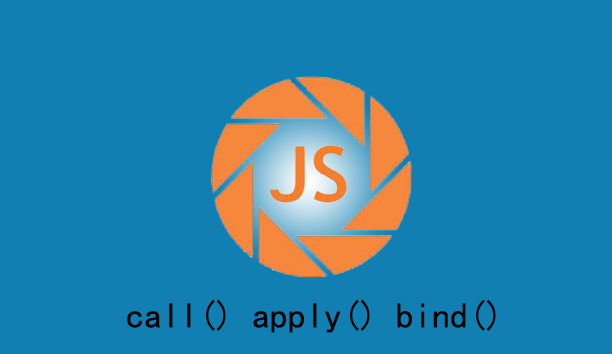﻿ 实例讲解js中的call() apply() bind()的用法和区别 – 码云笔记

# 实例讲解js中的call() apply() bind()的用法和区别

130℃

[隐藏]## 一、call()方法

`fun.call(thisArg[, arg1[, arg2[, ...]]])`

thisArg：fun函数运行时指定的this值，可能的值为：

· 不传，或者传null，undefined， this指向window对象

· 传递另一个函数的函数名fun2，this指向函数fun2的引用

· 值为原始值(数字，字符串，布尔值),this会指向该原始值的自动包装对象，如 String、Number、Boolean

· 传递一个对象，函数中的this指向这个对象

```function a(){

console.log(this);

}

function b(){}

a.call(b); // function b(){}```

```function test() {

// 将arguments转成数组

return Array.prototype.slice.call(arguments);

}

test(1,2,3);  // [1, 2, 3]```

## 二、apply()方法

// Chrome 14 以及 Internet Explorer 9 仍然不接受类数组对象。

// thisArg的可能值和call一样

fun.apply(thisArg[, argsArray])

```var numbers = [5, 6, 2, 3, 7];

var max = Math.max.apply(null, numbers);

console.log(max)  // 7```

## Call()、apply()的区别

call、apply的区别：作用完全一样，改变函数体内 this 的指向，只是接受参数的方式不一样。

```var func = function(arg1, arg2) {

};```

```func.call(this, arg1, arg2);

func.apply(this, [arg1, arg2])```

JavaScript 中，某个函数的参数数量是不固定的，因此要说适用条件的话，当你的参数是明确知道数量时用 call 。

bind：不立即执行。而apply、call 立即执行。

```数组之间追加
var array1 = [12 , "foo" , {name "Joe"} , -2458];

var array2 = ["Doe" , 555 , 100];

Array.prototype.push.apply(array1, array2);

/* array1 值为  [12 , "foo" , {name "Joe"} , -2458 , "Doe" , 555 , 100] */```
```获取数组中的最大值和最小值
var  numbers = [5, 458 , 120 , -215 ];

var maxInNumbers = Math.max.apply(Math, numbers),   //458

maxInNumbers = Math.max.call(Math,5, 458 , 120 , -215); //458```

number 本身没有 max 方法，但是 Math 有，我们就可以借助 call 或者 apply 使用其方法。

```验证是否是数组（前提是toString()方法没有被重写过）
functionisArray(obj){

return Object.prototype.toString.call(obj) === '[object Array]' ;

}```
```类（伪）数组使用数组方法
var domNodes = Array.prototype.slice.call(document.getElementsByTagName("*"));```

avascript中存在一种名为伪数组的对象结构。比较特别的是 arguments 对象，还有像调用 getElementsByTagName , document.childNodes 之类的，它们返回NodeList对象都属于伪数组。不能应用 Array下的 push , pop 等方法。

```function log(msg)　{

console.log(msg);

}

log(1);    //1

log(1,2);    //1```

```function log(){

console.log.apply(console, arguments);

};

log(1);    //1

log(1,2);    //1 2```

`log("hello world");    //(app)hello world`

```function log(){

var args = Array.prototype.slice.call(arguments);

args.unshift('(app)');

console.log.apply(console, args);

};```

bind 详解

MDN的解释是：bind()方法会创建一个新函数，称为绑定函数，当调用这个绑定函数时，绑定函数会以创建它时传入 bind()方法的第一个参数作为 this，传入 bind() 方法的第二个以及以后的参数加上绑定函数运行时本身的参数按照顺序作为原函数的参数来调用原函数。

```var foo = {

bar : 1,

eventBind: function(){

var _this = this;

\$('.someClass').on('click',function(event) {

/* Act on the event */

console.log(_this.bar);     //1

});

}

}```

```var foo = {

bar : 1,

eventBind: function(){

\$('.someClass').on('click',function(event) {

/* Act on the event */

console.log(this.bar);      //1

}.bind(this));

}

}```

```var bar = function(){

console.log(this.x);

}

var foo = {

x:3

}

bar(); // undefined

var func = bar.bind(foo);

func(); // 3```

```var bar = function(){

console.log(this.x);

}

var foo = {

x:3

}

var sed = {

x:4

}

var func = bar.bind(foo).bind(sed);

func(); //?

var fiv = {

x:5

}

var func = bar.bind(foo).bind(sed).bind(fiv);

func(); //?```

## apply、call、bind比较

```var obj = {

x: 81,

};

var foo = {

getX: function() {

return this.x;

}

}

console.log(foo.getX.bind(obj)());  //81

console.log(foo.getX.call(obj));    //81

console.log(foo.getX.apply(obj));   //81```

apply 、 call 、bind 三者都是用来改变函数的this对象的指向的；

apply 、 call 、bind 三者第一个参数都是this要指向的对象，也就是想指定的上下文；

apply 、 call 、bind 三者都可以利用后续参数传参；